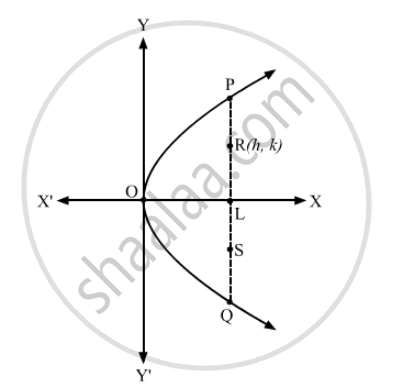Advertisement Remove all ads

# The Locus of the Points of Trisection of the Double Ordinates of a Parabola is a - Mathematics

MCQ

The locus of the points of trisection of the double ordinates of a parabola is a

#### Options

• pair of lines

•  circle

• parabola

• straight line

Advertisement Remove all ads

#### Solution

parabolaSuppose PQ is a double ordinate of the parabola $y^2 = 4ax$

Let R and be the points of trisection of the double ordinates.
Let $\left( h, k \right)$ be the coordinates of R.

Then, we have:
OL = h  and RL = k

$\therefore RS = RL + LS = k + k = 2k$
$\Rightarrow PR = RS = SQ = 2k$
$\Rightarrow LP = LR + RP = k + 2k = 3k$

Thus, the coordinates of P are $\left( h, 3k \right)$ which lie on $y^2 = 4ax$

∴ $9 k^2 = 4ah$

Hence, the locus of the point (hk) is $9 y^2 = 4ax$  i.e.  $y^2 = \left( \frac{4a}{9} \right)x$ which represents a parabola.

Is there an error in this question or solution?
Advertisement Remove all ads

#### APPEARS IN

RD Sharma Class 11 Mathematics Textbook
Chapter 25 Parabola
Q 7 | Page 29
Advertisement Remove all ads

#### Video TutorialsVIEW ALL 

Advertisement Remove all ads
Share
Notifications

View all notifications

Forgot password?